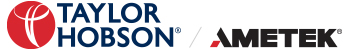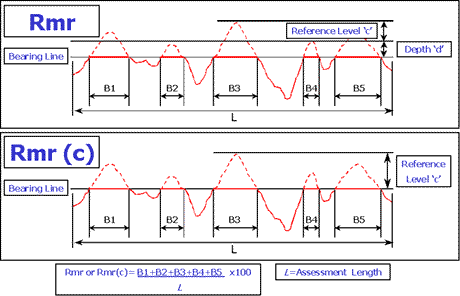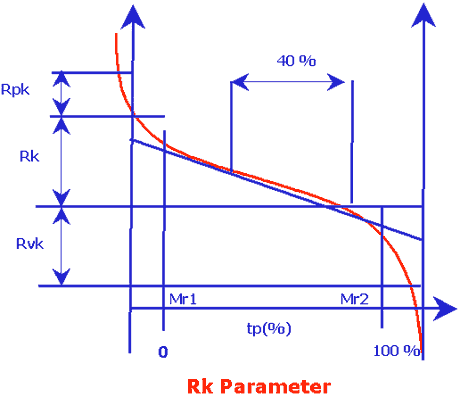Skip to content

# What are Hybrid Parameters?

## Hybrid Parameters:

Hybrid Parameters are defined as the combinations of spacing and amplitude parameters - Rdq, Wdq, Pdq, Rλq, Wλq, Pλq are more useful than a parameter based solely on amplitude or spacing. Rdq (RΔq, Rdelq) is the rms slope of the profile within the sample length.

This parameter is very useful for assessing reflectivity, friction, adhesion, vibration etc and is a measurement of the angular slope of the profile. Results are given in degrees. Being a hybrid parameter (h parameter), determined from both amplitude and spacing information, it is, for some applications. Generally, the lower the angle, the better the reflection, the higher the angle, the greater the friction. Rlq(Rλq, Rlamdaq) - is the root means square wavelength, a measure of the spacings between local peaks and valleys, taking into account their relative amplitudes and individual spatial frequencies.

Wdq, Wλq, Pdq and Pλq are the corresponding parameters from the waviness and primary profiles, respectively. Material Ratio Rmr is the length of material surface (expressed as a percentage of the evaluation length L) at a depth (d) below a reference level (c) Material Ratio Rmr (c) is the length of material surface (expressed as a percentage of the evaluation length L) at a depth (c) below the highest peak. Note: Most analysis packages allow the reference line for Rmr to be set from various levels such as using the mean line, the lowest valley, the highest peak or even from a material ratio level.Rpk, Rk, Rvk, MR1, MR2. These parameters were specifically designed for the control of the potential wear in cylinder bores in the automotive industry, They attempt to describe in numeric terms the wear characteristics of the bore by use of a material ratio curve. The filter used to analyse Rk and its associated parameters is a specific filter described in ISO 13 565 Part 1 (DIN 4776)Rpk - means Reduced Peak Height and is a measurement of the peaks of the surface in the cylinder bore. These peaks will be the areas of most rapid wear when the engine is first run. Rk - Kernal Roughness Depth or Core Roughness Depth - the long term running surface which will influence the performance and life of the cylinder. (Also the depth of the Roughness Core Profile or the load bearing area of the surface) Rvk (valley depth) is a measurement of the oil retaining capability of the valleys of the surface produced during the machining process (plateau honing).

Mr1 - is the Material Ratio corresponding to the upper limit position of the roughness core. (Where the Rpk and Rk depths meet on the material ratio curve.) Mr2 - is the Material Ratio corresponding to the lower limit position of the roughness core. (Where the Rvk and Rk depths meet on the material ratio curve) A1 - is the 'peak area' of the material ratio curve. It is calculated as the area of a right angled triangle of base length 0% to Mr1 and height Rpk. A2 - is the 'valley area' of the material ratio curve. It is calculated as the area of a right angled triangle of base length Mr2 to 100% and height Rvk